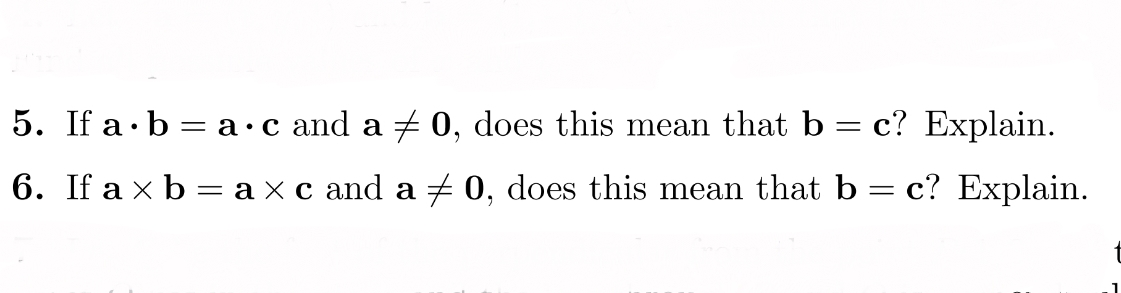# 5. If a.b-a-c and a f 0, d . 6. If ax b-ax c and aメ0, does this mean that b-c? Explain. oes this mean that bc. Explain

Questionhelp_outlineImage Transcriptionclose5. If a.b-a-c and a f 0, d . 6. If ax b-ax c and aメ0, does this mean that b-c? Explain. oes this mean that bc. Explain fullscreen

### Want to see the step-by-step answer?